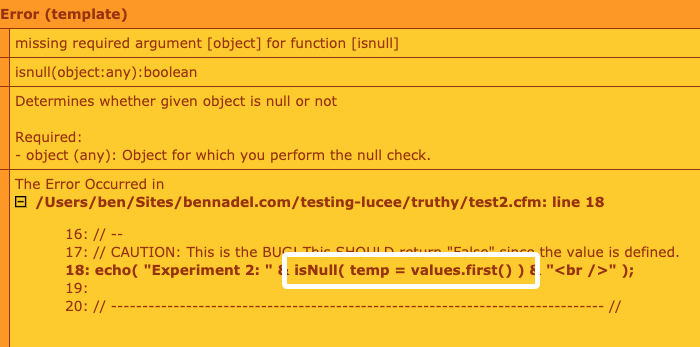# Possible Bug: Variable Assignment Gets Swallowed By Function Invocation In Lucee 5.3.2.77

By on
Tags:

UPDATE: On Twitter, John Pansewicz pointed out why this doesn't work. And, boy do I feel foolish. This "assignment" syntax that I'm trying to use in the context of a Function call is actually the named parameters invocation of that Function. So, I'm not assigning the variable, I'm invoking the Function with the given key-value pair.

I'm such a doofus :P

A couple of days ago, I celebrated the fact that I could finally embed an assignment operation inside of other expressions within Lucee 5. And, while the example that I demonstrated worked, it turns out that this is not fully supported feature in all contexts. Specifically, I am running into a strange bug in which variable assignment appears to get "swallowed" or "suppressed" when it is in the midst of a Function invocation in Lucee 5.3.2.77.

To see this in action, we can assign the result of an `array.first()` call while also checking to see if the value is null:

``````<cfscript>

values = [ "a", "b" ];

// Control case so that we demonstrate that .first() will return a non-null value
// when called multiple times in a row.
echo( "Control 1: " & values.first() & "<br />" );
echo( "Control 2: " & values.first() & "<br />" );

// An experiment to see if we can ASSIGN the value AND RETURN it from an expression
// at the same time.
echo( "Experiment 1: " &  ( temp = values.first() ) & "<br />" );

// Another experiment to see if we an ASSIGN the value AND PASS IT into a function
// call at the same time.
// --
// CAUTION: This is the BUG! This SHOULD return "False" since the value is defined.
echo( "Experiment 2: " &  isNullWrapper( temp = values.first() ) & "<br />" );

// ------------------------------------------------------------------------------- //
// ------------------------------------------------------------------------------- //

// NOTE: I am using a UDF wrapper for isNull() because if I try to use isNull()
// directly in this experiment, I get the following error:
// --
// missing required argument [object] for function [isnull]
// --
// For some reason, this issue is side-stepped if I wrap the isNull() call inside of
// a custom function.
public boolean function isNullWrapper( any value ) {

return( isNull( value ) );

}

</cfscript>
``````

In the first two lines, we are just confirming that we can call `array.first()` several times in a row and get the same value (ie, that the act of calling `.first()` isn't somehow affecting subsequent calls to `.first()`).

In the third line, we are then testing to see if we can call `array.first()` and assign the value at the same time (this works).

Then, in the fourth line, we are testing to see if we can call `array.first()` and assign the value as part of a larger Function call for `isNullWrapper()`.

Now, if we run this Lucee CFML code, we get the following output:

Control 1: a
Control 2: a
Experiment 1: a
Experiment 2: true

As you can see, Experiment 2 incorrectly came back as true, when it should have been false (since the `.first()` call returns a non-null value). In fact, I had to wrap the `isNull()` call inside of a custom Function since trying to do this as part of an `isNull()` call was throwing an error:As you can see from the screenshot, if I try to perform the assignment as part of the expression:

isNull( temp = values.first() )

... Lucee thinks that I'm attempting to call `isNull()` with zero arguments.

Now, I can use assignment as part of a larger expression, I just can't use it as part of a Function call at this time. To demonstrate, we can successfully use the assignment as part of a `while()` condition:

``````<cfscript>

values = [ 3, 29, 0, 12, 4 ];
valueIndex = 0;

// Notice that we CAN USE ASSIGNMENT inside of a larger EXPRESSION as long as the
// parent expressions is NOT THE ARGUMENT to a Function invocation.
while ( value = values[ ++valueIndex ] ) {

echo( "Value: #value# <br />" );

}

</cfscript>
``````

In this case, we're relying on the fact that the values in the array can be implicitly cast to Boolean. As such, when we run this Lucee ColdFusion code, we get the following expected output:

Value: 3
Value: 29

Notice that once the value-assignment set `value` to `0`, the `while()` condition was no longer true and the loop ended.

Clearly, we can still use assignment operations as part of a larger expression. This is evident in my previous post as well as the `while()` example above. But, we can't perform an assignment and call a Function at the same time, not in Lucee 5.3.2.77. I intend to file this as a bug.

Want to use code from this post? Check out the license.Off topic (sorry), but I've noticed you wrap all your return statements in parenthesis (e.g. `return( isNull( value ) );`). Does this guard against certain edge case behavior, making it a best practice? Or is this personal preference?@All,

Sorry -- I'm a doofus -- when using the `=` in the context of a Function, this is actually method invocation named parameters. As such, this is doing what it is supposed to do.

I was so focused on the syntax that I didn't even think about the Function all.